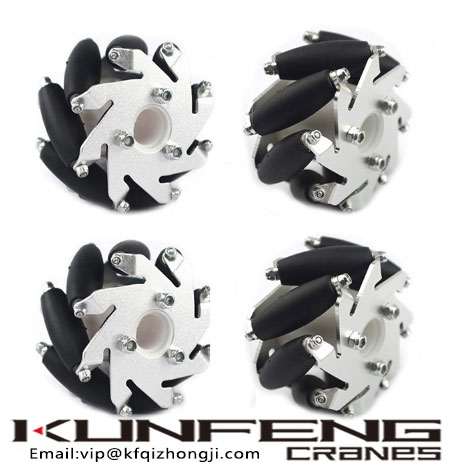Email:vip@kfqizhongji.com

# About forward and reverse kinematics model of Mecanum wheel chassis

Forward and inverse kinematics model of Mecanum wheel .
Taking the O-rectangular installation as an example, the landing positions of the four wheels form a rectangle. The forward kinematic model will get a series of formulas that allow us to calculate the movement state of the chassis through the speed of the four wheels; the formula obtained by the inverse kinematic model is based on the chassis The state of motion is calculated to calculate the speed of the four wheels. It should be noted that the movement of the chassis can be described by three independent variables: X-axis translation, Y-axis translation, and yaw rotation; and the speed of the four wheat wheels is also provided by four independent motors. Therefore, there is a certain constraint relationship between the reasonable speeds of the four wheat wheels. The inverse kinematics can obtain a unique solution, while the equations in positive kinematics that do not meet this constraint relationship will have no solution.
First try to build an inverse kinematics model. Because the mathematical model of the wheat wheel chassis is more complicated, we will perform it in four steps:
① Describe the movement of the chassis into three independent variables to describe;
② According to the result of the first step, calculate the speed of the axis position of each wheel;
③ According to the result of the second step, calculate the speed of each roller that is in contact with the ground;
④ According to the results of the third part, calculate the true speed of the wheels.